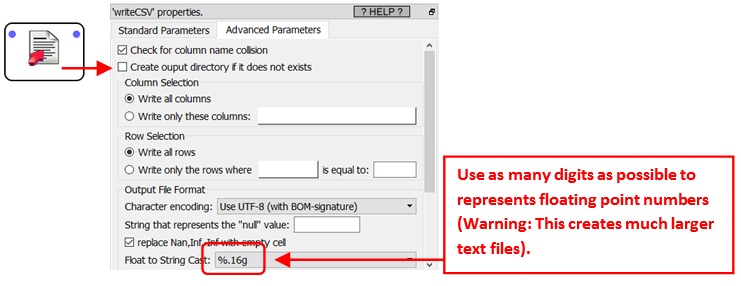Navigation:  »No topics above this level«

Typically, the “INSERT” operations are very slow inside a database and incurs a high load on the database system. So, most database vendors offer specialized tools called "bulk upload" that allow you to do many “INSERT” at very high speed and at a lower processing cost. Typically, these "bulk upload" tools take as input large text files and copy them into the database. There exists two different problems when working with text files:

1.Text file cannot store the “NULL” value (that is represented inside the Anatella-data-preview-window by an empty cell with a *red* background): Text files can only store strings of zero-length (that is represented inside the Anatella-data-preview-window by an empty cell with a *white* background). Thus, you might lose some valuable information here (because, from the point-of-view of predictive modeling, the NULL value might represent a concept fundamentally different than the “” value).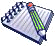In opposition to classical “Bulk Upload” tools, the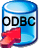Upsert Action and the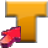TeradataWriter Action are both able to safely send to the database NULL values.

2.Storing floating-point numbers inside a text file is the most common source of many large “rounding errors”: i.e. You’ll get a slight loss of precision or accuracy when storing floating-point numbers inside text files because of the decimal↔binary convertion: See the next paragraph for more details on this subject. When these "rounding errors" accumulate, they can represent a large quantity of Euros/Dollars (There was even a movie about this subject: i.e. A coder that recovered the money losts inside the "rounding errors" (several millions euros) and put them on his own bank account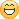)

Let’s now talk about how the floating-point numbers are stored inside a computer. Typically, the floating-point numbers are stored & manipulated in binary notation: 010011101 (more details about this subject here and here: IEEE 754-1985). Unfortunately, in text files, these same numbers are stored in decimal notation: e.g. the number Pi is 3.14159265458, 10, and so on. Converting fractional (ie non-integer) numbers from decimal notation to binary notation (and in the other direction: from binary notation to decimal notation) is a (significant) source of rounding error.The most well-known example of "rounding error" due to the convertion from decimal to binary notation is "1-0.9-0.1" that does not give 0 as output as expected (it gives 2e-17 instead).

The conversion from decimal notation (used by humans to represent numbers inside a text) to binary notations (the way the numbers are manipulated and stored inside a computer) is usually the most common and most important source of "rounding errors" in a computer program. Hopefully for integer numbers, we don't loose any precision during the conversion.Anatella tries its best to avoid any "rounding errors" and keeps the floating-point numbers stored in binary representation at all the time. For example, when Anatella connects to a database through ODBC or OleDB, it always tries to receive the floating-point numbers directly in binary notation (to avoid the "bad" conversion from/to decimal notation). In the same spirit, when Anatella make some "INSERT" into a database (using theUpsert Action or theTeradataWriter Action), it also sends to the database the floating-point numbers in Binary notation, as bits&bytes (without converting them to text: i.e. there is no conversion to decimal notation).

This means that, with Anatella, you can safely "copy" floating-point numbers from one database to another database without any loss of accuracy or precision because all the data is processed "in binary" (i.e. there is no convertion to decimal notation) (i.e. in the data preview window, the columns are all on a "blue background"). This ensure a total precision: i.e. 0% loss of accuracy. With most other ETLs (e.g. ETL in Java), you always have a loss of accuracy.

When you are using standard "bulk upload" tools, you'll lose some precision on the floating-point numbers because these tools expect text files as input (and inside the text files, all the floating-point numbers are converted to decimal notation). To reduce to the minimum this loss of precision, you should instruct Anatella to use as many digits as possible to represents floating-point numbers in the text file: This is done using this parameter inside the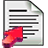writeCSV Action: